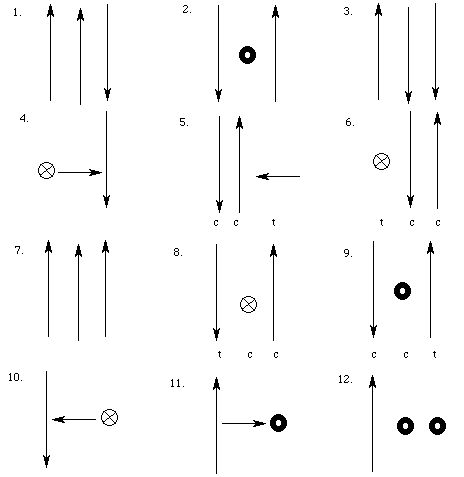# Problems for Chapter 5

5-1 through 5-12. For each of the following current configurations, specify the direction of the magnetic field at the location of the test current due to the configuration currents, and indicate the direction of the forces which the test current will feel, if any. If the test current will rotate, specify the direction of rotation. Unless otherwise indicated, the outer two currents of each triplet are the configuration currents, and the middle current is the test current. Assume that all currents have the same value of I.5-13. Use the "Mass Spectroscopy" Mathematica notebook to "measure" the masses of the following ions: H +, Be ++, O --, Ni + and U ++. The notebook assumes a 1.2 T magnetic field and a velocity of .01 m / s.

## Need more problems about magnetic forces?

If you have stumbled on this page, and the equations look funny (or you just want to know where you are!), see the College Physics for Students of Biology and Chemistry home page.